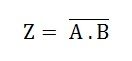# NAND Gate

The NAND gate is a combination of an AND gate and NOT gate. They are connected in cascade form. It is also called Negated And gate. The NAND gate provides the false or low output only when their outputs is high or true. The NAND gate is essential because different types of a boolean function are implemented by using it.

The NAND gate has the property of functional completeness. The function completeness means any types of gates can be implemented by using the NAND gate. It performs the function of OR, NOR and AND gate.

The logic symbol for the gate is shown below:The logic circuit of the NAND gate is shown below:From the logic circuit, the output can be expressed as:The equation is read as “Z equals NOT A AND B”. Since the logic circuit involves an AND gate followed by an inverter. The output can only be low when both the inputs are high.

The truth table of the NAND gate is given below:

ABZ
001
011
101
110

From the truth table of the gate, it is clear that all the inputs must be high to get a low output and if any of the input is low, the output obtained will be high. If any one of the input is also high the output will be high that is 1.